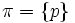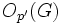# P-constrained group

The article defines a property of groups, where the definition may be in terms of a particular prime that serves as parameter
View other prime-parametrized group properties | View other group properties

## Definition

### Definition for a general finite group

Let$G$ be a finite group and$p$ be a prime number. We say that$G$ is$p$-constrained if the following is true for one (and hence, any)$p$-Sylow subgroup of$G$:$C_G(P \cap O_{p',p}(G)) \le O_{p',p}(G)$.

Here,$C_G(P)$ denotes the centralizer of$P$ in$G$.$O_{p',p}$ is the second member of the lower pi-series for$\pi = \{ p \}$.

### Definition for a p'-core-free finite group

This is the same as the previous definition, restricted to p'-core-free groups.

Let$G$ be a finite group and$p$ be a prime number. Suppose further that the p'-core of$G$ is trivial, i.e.,$O_{p'}(G)$ is the trivial group. Equivalently, every nontrivial normal subgroup of$G$ has order divisible by$p$. Then, we say that$G$ is$p$-constrained if its p-core is a self-centralizing subgroup, i.e.,:$\! C_G(O_p(G)) \le O_p(G)$

### Equivalence of definitions and its significance

Further information: equivalence of definitions of p-constrained group

It turns out that, from the above definitions:$G$ is$p$-constrained$\iff$$G/O_{p'}(G)$ is$p$-constrained.

This allows us to define$p$-constraint for arbitrary finite groups in terms of$p$-constraint for$p'$-core-free finite groups.

## Relation with other properties

### Stronger properties

Property Meaning Proof of implication Proof of strictness (reverse implication failure) Intermediate notions
strongly p-solvable group
p-solvable group p-solvable implies p-constrained p-constrained not implies p-solvable
finite solvable group (via p-solvable) (via p-solvable)
p-nilpotent group (via p-solvable) (via p-solvable)
finite nilpotent group (via finite solvable) (via finite solvable)

### Incomparable properties

Property Meaning Proof of one non-implication Proof of other non-implication
p-stable group p-constrained not implies p-stable p-stable not implies p-constrained
group of Glauberman type for a prime p-constrained not implies Glauberman type Glauberman type not implies p-constrained

## Metaproperties

Metaproperty name Satisfied? Proof Statement with symbols
subgroup-closed group property No p-constraint is not subgroup-closed It is possible to have a finite group$G$, a subgroup$H$, and a prime number$p$ such that$G$ is$p$-constrained and$H$ is not.
quotient-closed group property No p-constraint is not quotient-closed It is possible to have a finite group$G$, a normal subgroup$N$, and a prime number$p$ such that$G$ is$p$-constrained and$G/N$ is not.